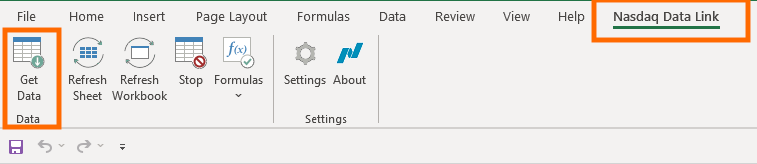Note that each data feed on Nasdaq Data Link is delivered through one of the following Nasdaq data Link APIs:

• Streaming API for real-time data
• REST API for real-time or delayed data
• REST API for time-series
• REST API for tables

The Excel Add-in only works with data that is delivered through the REST API for time-series or the REST API for tables. The Excel Add-In works with Windows and the desktop versions of Excel 2013, 2016 and 2019. The Excel Add-In is not compatible with online versions of Excel, and it is not currently compatible with Mac.

Time-series
Once the Excel Add-In is installed, you can download time-series data by using the Nasdaq Data Link Formula Builder or by using the QSERIES formula

To use the Nasdaq Data Link Formula Builder, simply open Excel, go to the "Nasdaq Data Link" tab and click the "Get Data" button.Here is a short tutorial that explains how to use the Nasdaq Data Link Formula Builder with time-series data:

To learn how to use the QSERIES formula, see the documentation here.

Here is a short tutorial that explains how to use the QSERIES formula:

Tables
Once the Excel Add-In is installed, you can retrieve data from tables by using the Nasdaq Data Link Formula Builder or by using the QTABLE formula.

To use the Nasdaq Data Link Formula Builder, simply open Excel, go to the "Nasdaq Data Link" tab and click the "Get Data" button.Here is a short tutorial that explains how to use the Nasdaq Data Link Formula Builder with data from tables:

To learn how to use the QTABLE formula, see the documentation here.

Here is a short tutorial that explains how to use the QTABLE formula: